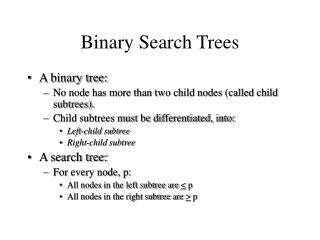DownloadDownload PresentationBinary Search Trees

# Binary Search Trees

Télécharger la présentation## Binary Search Trees

- - - - - - - - - - - - - - - - - - - - - - - - - - - E N D - - - - - - - - - - - - - - - - - - - - - - - - - - -
##### Presentation Transcript

1. Binary Search Trees • A binary tree: • No node has more than two child nodes (called child subtrees). • Child subtrees must be differentiated, into: • Left-child subtree • Right-child subtree • A search tree: • For every node, p: • All nodes in the left subtree are < p • All nodes in the right subtree are > p

2. Binary Search Tree - Example

3. Binary Search Trees (cont) • Searching for a value is in a tree of N nodes is: • O(log N) if the tree is “balanced” • O(N) if the tree is “unbalanced”

4. “Unbalanced” Binary Search Trees • Below is a binary search tree that is NOT “balanced”

5. Properties of Binary Trees • A binary tree is a full binary tree if and only if: • Each non leaf node has exactly two child nodes • All leaf nodes have identical path length • It is called full since all possible node slots are occupied

6. A Full Binary Tree - Example

7. Full Binary Trees • A Full binary tree of height h will have how many leaves? • A Full binary tree of height h will have how many nodes?

8. Complete Binary Trees • A complete binary tree (of height h) satisfies the following conditions: • Level 0 to h-1 represent a fullbinary tree of height h-1 • One or more nodes in level h-1 may have 0, or 1 child nodes • If j,k are nodes in level h-1, then j has more child nodes than k if and only if j is to the left of k

9. Complete Binary Trees - Example

10. Complete Binary Trees (cont) • Given a set of N nodes, a complete binary tree of these nodes provides the maximum number of leaves with the minimal average path length (per node) • The complete binary tree containing n nodes must have at least one path from root to leaf of length log n

11. Height-balanced Binary Tree • A height-balanced binary tree is a binary tree such that: • The left & right subtrees for any given node differ in height by no more than one • Note: Each complete binary tree is a height-balanced binary tree

12. Height-balanced Binary Tree - Example

13. Advantages of Height-balanced Binary Trees • Height-balanced binary trees are “balanced” • Operations that run in time proportional to the height of the tree are O(log n), n the number of nodes with limited performance variance • Variance is a very important concern in real time applications, e.g. connecting calls in a telephone network